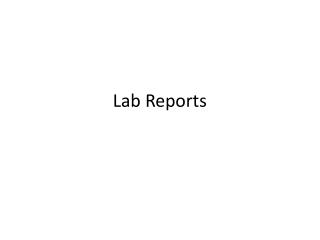DownloadDownload PresentationLab Reports

# Lab Reports

Download Presentation## Lab Reports

- - - - - - - - - - - - - - - - - - - - - - - - - - - E N D - - - - - - - - - - - - - - - - - - - - - - - - - - -
##### Presentation Transcript

1. Lab Reports

2. Agood lab report: • Contains all key elements from scientific method • Has an excellent hypothesis • Collects data accurately • Has thoughtful answers to analysis questions

3. Bond with a Classmate Purpose: •To understand how two elements bond to become an ionic compound Problem:How do ions form bonds with other ions? Hypothesis:(complete before you begin): Procedure: 1. Obtain one tag from the teacher. Are you a positive (+) or negative (-) ion? 2. Find an ion with the opposite charge. 3. In the data table, write your element symbol and charge, along with your partner’s element and charge. 4. Write the compound into the data table. Remember, the positive ion is written first. 6. Find a new partner. 7. After your 5th bond, get a new tag and repeat steps 1 – 7. Do this 2 times (total of 10) Data Chart Analysis Questions: 1. What is a compound? 2. What is an ionic compound? 3. Why can’t two negative ions or two positive ions bond together? Explain. 4. How are ionic compounds named? Conclusion Statement: Write a conclusion that identifies why/why not your hypothesis was correct

4. Scientific Method Reminder • Remember, the scientific method has 6 steps, these are in your lab report • 1. Ask A Question (Problem) • 2. Make a Hypothesis (Hypothesis) • 3. Conduct an experiment (Procedure) • 4. Analyze Data (Data Tables & Analysis Questions) • 5. Draw a conclusion (Conclusion Statement) • 6. Report Results (Turn in your Report!!)

5. What is a HYPOTHESIS? A hypothesis is a tentative statement that proposes a possible explanation to some phenomenon or event. • A hypothesis is an answer to the problem (question) • A useful hypothesis is a testable statement which is based in science. • It is an If….then statement!

6. How do you write it? • Look at the Question (Problem)! • Figure out what the problem is asking 3. Write your hypothesis so that it answers the question appropriately 4. Use IF………THEN statements

7. Appropriate/Correct Questions • Questions that ask if something is appropriate or a good idea also must have a reason • Is measuring with your body a good system of measurement? • If I measure with my body, then I will make correct measurements because I always have my body with me.

8. HOW Questions • HOW: if a problem starts with How, then you must give a reason, not just a statement How do you determine relative densities of unknown liquids? If I layer the liquids, then I will be able to determine their relative densities.

9. Practice HOW Questions • How do you play football? • How do you know who your friends are? • How do you determine which music you like to listen to?

10. Practice Writing Hypotheses • Write the problem, then Write an appropriate Hypothesis for these problems: • Use If/Then statements • How do you determine which day of the week most students in the class are born? • Which is more successful studying for tests or not studying? • How can you tell if a substance is flammable? • What is the best method for arriving at school on time?

11. NEVER! • Turn in a lab report that does not have a hypothesis • Answer questions without complete sentences • Leave data charts blank • Write numbers without units • Use slang or write in colors, including the word “Well,” • Turn in a lab report that you are not proud of REMEMBER: LAB REPORTS COUNT AS TEST GRADES!